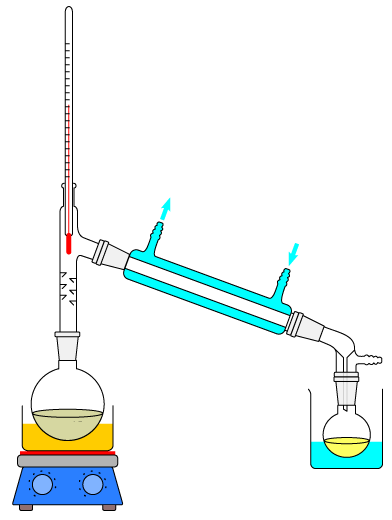10 minutes maximum! Can you do it in 5?

Q1-3 refer to the following particle diagrams. Different atoms are represented by orange and blue circles.Select the diagram which represents ...

1. ...a mixture of gases.
2. ...a gaseous compound.
3. ...chlorine gas (Cl2)

4. Which of the following is correct about diagrams X and Y?Diagram X represents.. Diagram Y represents.. A two elements a mixture B two elements a compound C two compounds a mixture D two compounds a compound
5. Which type of substance can be separated by filtration?
• A.   an element
• B.   a compound
• C.   a mixture
• D.   a molecule
6. This apparatus can be used to obtain pure water from sea water.What is the name of the process shown?

• A.   filtration
• B.   evaporation
• C.   crystallisation
• D.   distillation

Q7-9. Select the most suitable technique to obtain the first named substance from each of the following mixtures:

7. Chalk from a mixture of chalk and water.
8. Kerosene from crude oil.
9. Copper(II)sulfate from copper(II)sulfate solution.

10. A student investigated the colour in three different flowers X, Y and Z.

He set up the apparatus as shown:An error made by the student was he ...

• A. put a lid on the beaker
• B. drew the start line in ink
• C. put the start line above the water level
• D. put all three spots on the same piece of chromatography paper